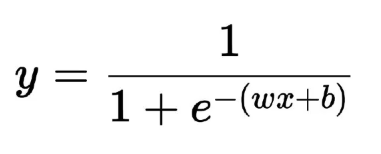# Cat vs Non-cat Classifier - Defining some utility functions - Sigmoid Function

As we know, we use sigmoid activation function in logistic regression to introduce non-linearity to the decision curve. All the negative extreme values will be squashed towards 0, while the positive extreme values will be squashed towards 1. The value of sigmoid(0) is 0.5.Note:

• `np.exp()` calculates the exponent value of each element of the input array.

• `np.linspace(a,b,n)` returns a NumPy array with n equally spaced elements, and `a` and `b` are respectively the first and last elements in the resultant array.

INSTRUCTIONS
• Let us write our own sigmoid function.

``````def sigmoid(z):
s = 1 / (1 + np.exp(-z))
return s
``````
• Now, let us use the above defined sigmoid function.

• Get 2000000 linearly spaced values between -10 and 10 using `np.linspace()` and store it in the variable `z`

``````z = << your code comes here >>
``````
• Call the `sigmoid` function by passing the `z`, and receive the returned value in `sigmoid_z` variable.

``````sigmoid_z = << your code comes here >>
``````
• Let us plot the `z` vs `sigmoid_z` values using matplotlib.

``````plt.plot(z, sigmoid_z)
plt.xlabel("z")
plt.ylabel("Sigmoid(z)")
plt.show()
``````

No hints are availble for this assesment

Answer is not availble for this assesment

Note - Having trouble with the assessment engine? Follow the steps listed here